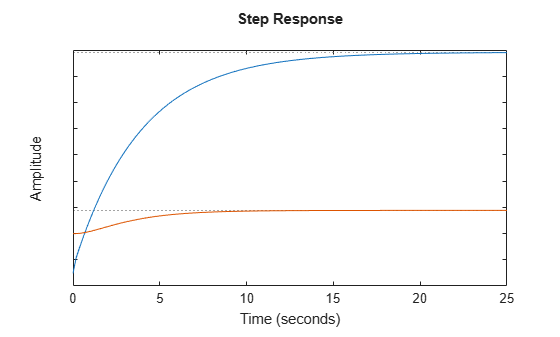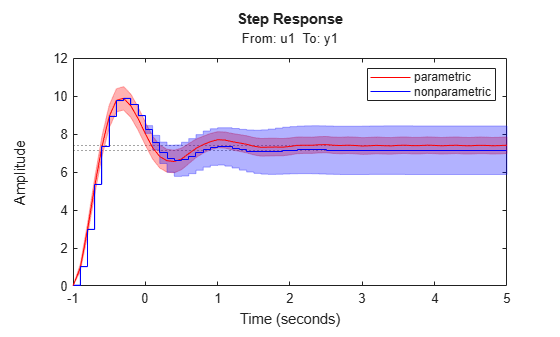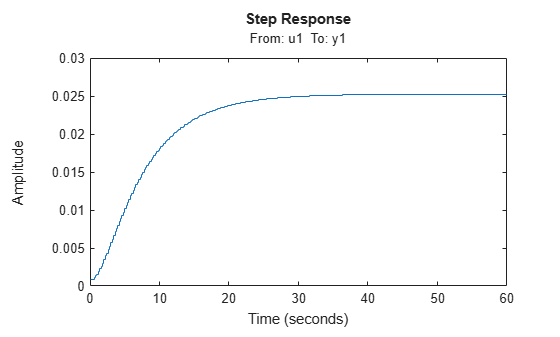stepplot

Plot step response with additional plot customization options

Description

stepplot lets you plot dynamic system step responses with a broader range of plot customization options than step. You can use stepplot to obtain the plot handle and use it to customize the plot, such as modify the axes labels, limits and units. You can also use stepplot to draw a step response plot on an existing set of axes represented by an axes handle. To customize an existing step plot using the plot handle:

1. Obtain the plot handle

2. Use getoptions to obtain the option set

3. Update the plot using setoptions to modify the required options

For more information, see Customizing Response Plots from the Command Line. To create step plots with default options or to extract step response data, use step.

example

h = stepplot(sys) plots the step response of the dynamic system model sys and returns the plot handle h to the plot. You can use this handle h to customize the plot with the getoptions and setoptions commands.

example

h = stepplot(sys1,sys2,...,sysN) plots the step response of multiple dynamic systems sys1,sys2,…,sysN on the same plot. All systems must have the same number of inputs and outputs to use this syntax.

example

h = stepplot(sys1,LineSpec1,...,sysN,LineSpecN) sets the line style, marker type, and color for the step response of each system. All systems must have the same number of inputs and outputs to use this syntax.

example

h = stepplot(___,tFinal) simulates the step response from t = 0 to the final time t = tFinal. Specify tFinal in the system time units, specified in the TimeUnit property of sys. For discrete-time systems with unspecified sample time (Ts = -1), stepplot interprets tFinal as the number of sampling intervals to simulate.

example

h = stepplot(___,t) simulates the step response using the time vector t. Specify t in the system time units, specified in the TimeUnit property of sys.

h = stepplot(AX,___) plots the step response on the Axes object in the current figure with the handle AX.

example

h = stepplot(___,plotoptions) plots the step response with the options set specified in plotoptions. You can use these options to customize the step plot appearance using the command line. Settings you specify in plotoptions overrides the preference settings in the MATLAB® session in which you run stepplot. Therefore, this syntax is useful when you want to write a script to generate multiple plots that look the same regardless of the local preferences.

example

h = stepplot(___,dataoptions) plots the step response with the options set specified in dataoptions. You can use this syntax to specify options such as the step amplitude and input offset using the options set dataoptions. This syntax is useful when you want to write a script to generate multiple plots with the same option set. Use stepDataOptions to create the options set.

Examples

collapse all

For this example, use the plot handle to change the time units to minutes and turn on the grid.

Generate a random state-space model with 5 states and create the step response plot with plot handle h.

rng("default")
h = stepplot(sys);

Change the time units to minutes and turn on the grid. To do so, edit properties of the plot handle, h using setoptions.

setoptions(h,'TimeUnits','minutes','Grid','on');The step plot automatically updates when you call setoptions.

Alternatively, you can also use the timeoptions command to specify the required plot options. First, create an options set based on the toolbox preferences.

plotoptions = timeoptions('cstprefs');

Change properties of the options set by setting the time units to minutes and enabling the grid.

plotoptions.TimeUnits = 'minutes';
plotoptions.Grid = 'on';
stepplot(sys,plotoptions);You can use the same option set to create multiple step plots with the same customization. Depending on your own toolbox preferences, the plot you obtain might look different from this plot. Only the properties that you set explicitly, in this example TimeUnits and Grid, override the toolbox preferences.

Generate a step response plot for two dynamic systems.

h = stepplot(sys1,sys2);Each step response settles at a different steady-state value. Use the plot handle to normalize the plotted response.

setoptions(h,'Normalize','on')Now, the responses settle at the same value expressed in arbitrary units.

Compare the step response of a parametric identified model to a nonparametric (empirical) model, and view their 3-σ confidence regions. (Identified models require System Identification Toolbox™ software.)

Identify a parametric and a nonparametric model from sample data.

sys1 = ssest(z1,4);
sys2 = impulseest(z1);

Plot the step responses of both identified models. Use the plot handle to display the 3-σ confidence regions.

t = -1:0.1:5;
h = stepplot(sys1,'r',sys2,'b',t);
showConfidence(h,3)
legend('parametric','nonparametric')The nonparametric model sys2 shows higher uncertainty.

For this example, examine the step response of the following zero-pole-gain model and limit the step plot to tFinal = 15 s. Use 15-point blue text for the title. This plot should look the same, regardless of the preferences of the MATLAB session in which it is generated.

sys = zpk(-1,[-0.2+3j,-0.2-3j],1)*tf([1 1],[1 0.05]);
tFinal = 15;

First, create a default options set using timeoptions.

plotoptions = timeoptions;

Next change the required properties of the options set plotoptions.

plotoptions.Title.FontSize = 15;
plotoptions.Title.Color = [0 0 1];

Now, create the step response plot using the options set plotoptions.

h = stepplot(sys,tFinal,plotoptions);Because plotoptions begins with a fixed set of options, the plot result is independent of the toolbox preferences of the MATLAB session.

Load data for estimating a nonlinear Hammerstein-Wiener model.

z = iddata(y,u,0.2,'Name','Two tank system');

z is an iddata object that stores the input-output estimation data.

Estimate a Hammerstein-Wiener Model of order [1 5 3] using the estimation data. Specify the input nonlinearity as piecewise linear and output nonlinearity as one-dimensional polynomial.

sys = nlhw(z,[1 5 3],idPiecewiseLinear,idPolynomial1D);

Create an option set to specify input offset and step amplitude level.

opt = stepDataOptions('InputOffset',2,'StepAmplitude',0.5);

Plot the step response until 60 seconds using the specified options.

stepplot(sys,60,opt);Input Arguments

collapse all

Dynamic system, specified as a SISO or MIMO dynamic system model or array of dynamic system models. Dynamic systems that you can use include:

• Continuous-time or discrete-time numeric LTI models, such as tf, zpk, or ss models.

• Sparse state-space models, such as sparss or mechss models. Final time tFinal must be specified when using sparse models.

• Generalized or uncertain LTI models such as genss or uss (Robust Control Toolbox) models. (Using uncertain models requires Robust Control Toolbox™ software.)

• For tunable control design blocks, the function evaluates the model at its current value to plot the step response data.

• For uncertain control design blocks, the function plots the nominal value and random samples of the model.

• Identified LTI models, such as idtf (System Identification Toolbox), idss (System Identification Toolbox), or idproc (System Identification Toolbox) models. (Using identified models requires System Identification Toolbox™ software.)

If sys is an array of models, the function plots the step response of all models in the array on the same axes.

Line style, marker, and color, specified as a character vector or string containing symbols. The symbols can appear in any order. You do not need to specify all three characteristics (line style, marker, and color). For example, if you omit the line style and specify the marker, then the plot shows only the marker and no line.

Example: '--or' is a red dashed line with circle markers

Line StyleDescription
-Solid line
--Dashed line
:Dotted line
-.Dash-dot line
MarkerDescription
'o'Circle
'+'Plus sign
'*'Asterisk
'.'Point
'x'Cross
'_'Horizontal line
'|'Vertical line
's'Square
'd'Diamond
'^'Upward-pointing triangle
'v'Downward-pointing triangle
'>'Right-pointing triangle
'<'Left-pointing triangle
'p'Pentagram
'h'Hexagram
ColorDescription

y

yellow

m

magenta

c

cyan

r

red

g

green

b

blue

w

white

k

black

Final time for step response computation, specified as a scalar. Specify tFinal in the system time units, specified in the TimeUnit property of sys. For discrete-time systems with unspecified sample time (Ts = -1), stepplot interprets tFinal as the number of sampling intervals to simulate.

Time for step response simulation, specified as a vector. Specify the time vector t in the system time units, specified in the TimeUnit property of sys. The time vector must be real, finite, and must contain monotonically increasing and evenly spaced time samples.

The time vector t is:

• t = Tinitial:Tsample:Tfinal, for discrete-time systems.

• t = Tinitial:dt:Tfinal, for continuous-time systems. Here, dt is the sample time of a discrete approximation of the continuous-time system.

Target axes, specified as an Axes object. If you do not specify the axes and if the current axes are Cartesian axes, then stepplot plots on the current axes. Use AX to plot into specific axes when creating a step plot.

Step plot options set, specified as a TimePlotOptions object. You can use this option set to customize the step plot appearance. Use timeoptions to create the option set. Settings you specify in plotoptions overrides the preference settings in the MATLAB session in which you run stepplot. Therefore, plotoptions is useful when you want to write a script to generate multiple plots that look the same regardless of the local preferences.

For the list of available options, see timeoptions.

Step response data options set, specified as a step object. Specify options such as the step amplitude and input offset using the options set dataoptions. This is useful when you want to write a script to generate multiple plots with the same step amplitude and input offset values. Use stepDataOptions to create the options set.

Output Arguments

collapse all

Plot handle, returned as a handle object. Use the handle h to get and set the properties of the step plot using getoptions and setoptions. For the list of available options, see the Properties and Values Reference section in Customizing Response Plots from the Command Line.